# CBSE Solutions for Class 10 Maths

#### Select CBSE Solutions for class 10 Subject & Chapters Wise :

State quadratic equations have real roots or no real root :- −x2−2x−2=0

no real roots

State quadratic equations have real roots or no real root :-  x−1x−3=0, x≠0

real roots

State quadratic equations have real roots or no real root :- 2x2−6x+3=0

real roots

State quadratic equations have real roots or no real root :- −13x2+3x+7=0

real roots

State quadratic equations have real roots or no real root :- 11x2−12x−1=0

real roots

State quadratic equations have real roots or no real root :- 9x2+x+3=0

no real roots

State quadratic equations have real roots or no real root :- 3x2+6x+1=0

real roots

State quadratic equations have real roots or no real root :- sx2+x+7=0

no real roots

Find the roots of the quadratic equation using square method :-2x2−7x+3=0

2x2−7x+3=0

(x−7/4)2−(7/4)2+3/2=0
(x−7/4)2=49/16−3/2=0
(x−7/4)2=25/16
x−7/4=±5/4
or
x=1/2 or 3

Find the roots of the quadratic equation using factorization technique :-

x2−11x+30=0
Solution- x2−11x+30=0
x(x-5)-(x-6)=0
Roots are 5 and 6

Find the roots of the quadratic equation using factorization technique :-

x2−3x−10=0
Solution- x2−3x−10=0
x(x−5)+2(x−5)=0
(x+2)(x−5)=0
So roots are x=-2 and 5

Find whether statement is True or False  :- A quadratic equation can have at most 2 real roots

True

Find whether statement is True or False  :- The roots of the equation x2−1=0x2−1=0 are 1,-1

True

Find whether statement is True or False  :- There are no reals roots of the quadratic equation x2+4x+5=0x2+4x+5=0

True

In the roots of the following quadratic equation: 23x2 – 5x + 3 = 0 (2011D)We have, 2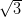x2 – 5x += 0
Here, a = 2, h = -5, c =D = b2 – 4ac
D = (-5)2 – 4 (2)()
= 25 – 24 = 1Solve for x: 4x2 – 4ax + (a2 – b2) = 0 (2011OD)

4x2 – 4ax + (a2 – b2) = 0
[4x2 – 4ax + a2] – b2 = 0
[(2x)2 – 2(2x)(a) + (a)2] – b2 = 0
(2x – a)2 – (b)2 = 0
(2x – a + b) (2x – a – b) = 0
2x – a + b = 1 or 2x – a – b = 0
2x = a – b or 2x = a + b

x =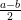or x =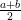Solve for x: 3x2 – 26x + 2 = 0 (2012D)

3x2 – 2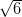x + 2 = 0
3x2 –x –x + 2 = 0x (x –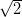–(x –) = 0
(x –)(x –) = 0x –= 0 x =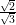x =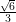….[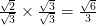Find the value(s) of k so that the quadratic equation 3x2 – 2kx + 12 = 0 has equal roots. (2012D)

Given: 3x2 – 2kx + 12 = 0
Here a = 3, b = -2k, c = 12
As the roots are equal

D = 0
b2 – 4ac = 0

(-2k)2 – 4(3) (12) = 0
4k2 – 144 = 0 k2 =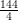= 36
k =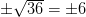Find the value(s) of k so that the quadratic equation 2x2 + kx + 3 = 0 has equal roots. (2012D)

Given: 2x2 + kx + 3 = 0
Here a = 2, b = k, c= 3
As the roots are equal

D = 0
As b2 – 4ac = 0
K2 – 4(2)(3) = 0
K2 – 24 = 0 or k2 = 24

k =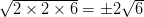Find the roots of the solution

 1    √3x2+10x−8√3=0 A    −7√3 and 7√7 2    √3x2+11x+6√3 =0 B    −√7 and 13√7/7 3    3√7x2+4x−√7=0 C    −3√3 and −2√3/3 4    √7x2−6x−13√7=0 D    2/√3 and −4√3

1-D, 2-C, 3-A, 4-B

Find the roots of the solution

 1    15x2−28=x A    −3√2 and 2√2. 2    4−11x=3x2 B    −1/16 and 1/3 3    48x2−13x−1=0 C    −4 and 1/3 4    x2+2√x−6=0 D    −4/3 and 7/5

1-D, 2-C, 3-B, 4-A

Find the roots of the solution

 1    6x2 + 11x + 3 = 0 A    25/4 and −4 2    6x2+x−12=0 B    −1/3 and −3/2 3    3x2−2x−1=0 C    4/3 and −3/2 4    4x2−9x=100 D    1 and −1/3

1-B, 2-C, 3-D, 4-A

Find the roots of the solution

 1    x2+6x+5=0 A    −5 and −7 2    9x2-3x-2=0 B    −1 and −5 3    x2+12x+35=0 C    7 and 11 4    x2=18x−77 D    -1/3 and 2/3

1-B, 2-D, 3-A, 4-C

Find the roots of the solution

 1    (2x − 3)(3x + 1) = 0 A    0 and -5/4 2    4x2 + 5x = 0 B    −9 and 9 3    3x2-243=0 C    3/2 and -1/3 4    2x2+x-6=0 D    -2 and -3/2

1-C, 2-A, 3-B, 4-D

### Chapter 4 : Quadratic Equations

In a quadratic equation, x represents an unknown form and a, b, c are the known values. An equation to be quadratic “a” should not be equal to 0. The equation is of the form ax2 + bx + c = 0. The values of a, b, and c are always real numbers. A quadratic equation can be calculated by completing the square. A quadratic equation has:
• Two different real roots.
• No real roots.
• Two equal roots.

### Browse & Download CBSE Books For Class 10 - All Subjects

The GSEB Books for class 10 are designed as per the syllabus followed Gujarat Secondary and Higher Secondary Education Board provides key detailed, and a through solutions to all the questions relating to the GSEB textbooks.

The purpose is to provide help to the students with their homework, preparing for the examinations and personal learning. These books are very helpful for the preparation of examination.

For more details about the GSEB books for Class 10, you can access the PDF which is as in the above given links for the same.